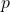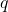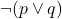# Prepositional Logic – Negation of Statements

From the previous article, you know that a prepositional statement can have truth value and the values are boolean – true or false. A statement having true value can be negated using ‘not‘ operator and then its value becomes false or vice-versa.

Consider the following example where variableis the statement “I am eating“.

\begin{aligned}
&p: "I \hspace{5px}am \hspace{5px} eating" \\\\
&negation \hspace{5px} of \hspace{5px} p \hspace{5px} or \hspace{5px} \neg p: " It \hspace{5px} is \hspace{5px}not \hspace{5px} the\hspace{5px} case \hspace{5px}that \hspace{5px}I \hspace{5px}am \hspace{5px}eating." \\
&or \\
&"I \hspace{5px}am \hspace{5px}not \hspace{5px} eating"
\end{aligned}

You can write a negative statement but a true statement and negate it too. Letbe the statement “I am not eating”.

\begin{aligned}
&q: "I \hspace{5px} am \hspace{5px} not \hspace{5px}eating"\\\\
&\neg q: "I \hspace{5px} am \hspace{5px}eating".
\end{aligned}

Given a compound preposition, a negation must be simplified first before any other logical operator. Its precedence is highest unless you have part of an expression enclosed in parenthesis.

\begin{aligned}
&1. \hspace{3px}\neg(p \lor q) \hspace{3px} and \hspace{3px} (q \lor \neg q)\\\\
&2. \hspace{3px}(p \lor p)\land \neg q
\end{aligned}

In the compound statement (1) there are two groups of parenthesis where first grouphas a negation. Therefore, we must evaluate the first group and then second group of parenthesis.

The expression (2) has only one parenthesis that must be evaluated first because cannot simplify the not operator with one variable.

### Truth Table for Negation

The negation operator is unary operator which give opposite results to a boolean value. The truth table gives all possible output for a given input combination and negation operator.